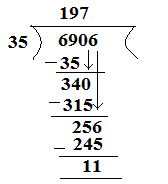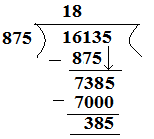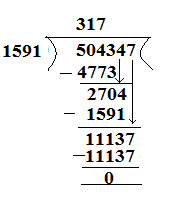Click to Chat

1800-1023-196

+91-120-4616500

CART 0

• 0

MY CART (5)

Use Coupon: CART20 and get 20% off on all online Study Material

ITEM
DETAILS
MRP
DISCOUNT
FINAL PRICE
Total Price: Rs.

There are no items in this cart.
Continue Shopping• Complete JEE Main/Advanced Course and Test Series
• OFFERED PRICE: Rs. 15,900
• View Details

```Chapter 4: Operations on Whole Numbers – Exercise 4.4

Question: 1

Does there exists a whole number ‘a’ such that a/a = a?

Solution:

Yes, there exists a whole number ‘a’ such that a/a = a.

The whole number is 1 such that,

1/1 = 1

Question: 2

Find the value of:

Solution:

(i) 23457 / 1 = 23457

(ii) 0 / 97 = 0

(iii) 476 + (840 / 84) = 476 + 10 = 486

(iv) 964 – (425 / 425) = 964 – 1 = 963

(v) (2758 / 2758) – (2758 + 2758) = 1 – 1 = 0

(vi) 72450 / (583 – 58) = 72450 + 525 = 138

Question: 3

Which of the following statements are true:

Solution:

(i) False

LHS: 10 / (5 × 2)

= 10 / 10

=1

RHS: (10 / 5) × (10 / 2)

= 2 × 5 = 10

(ii) True

LHS: (35 – 14) / 7

= 21 / 7

=3

RHS: 35 / 7 – 14 / 7

= 5 – 2 = 3

(iii) False

LHS: 35 – 14 / 7

= 35 – 2 = 33

RHS: 35 / 7 – 14 / 7

= 5 – 2

= 3

(iv) False

LHS: (20 – 5) / 5

= 15 / 5

= 3

RHS: 20 / 5 – 5

= 4 – 5 = -1

(v) False

LHS: 12 × (14 / 7)

= 12 × 2

= 24

RHS: (12 × 14) / (12 × 7)

= 168 / 84

=2

(vi) True

LHS: (20 / 5) / 2

= 4 / 2

=2

RHS : ( 20 / 2 ) / 5

= 10 / 5

= 2

Question: 4

Divide and check the quotient and remainder:

Solution:

(i) 7777 / 58 = 134Verification: [Dividend = Divisor × Quotient + Remainder ]

7772 = 58 × 134 + 0

7772 = 7772

LHS = RHS

(ii) 6906/35 gives quotient = 197 and remainder = 11Verification: [Dividend = Divisor × Quotient + Remainder ]

6906 = 35 × 197 + 11

6906 = 6895 + 11

6906 = 6906

LHS = RHS

(iii) 16135 / 875 gives quotient = 18 and remainder = 385.Verification: [Dividend = Divisor × Quotient + Remainder ]

16135 = 875 × 18 + 385

16135 = 15750 + 385

16135 = 16135

LHS = RHS

(iv) 16025/1000 gives quotient and remainder = 25Verification: [Dividend = Divisor × Quotient + Remainder ]

16025 = 1000 × 16 + 25

16025 = 16000 + 25

16025 = 16025

LHS = RHS

Question: 5

Find a number which when divided by 35 gives the quotient 20 and remainder 18.

Solution:

Dividend = Divisor × Quotient + Remainder

Dividend = 35 × 20 + 18

= 700 + 18

= 718

Question: 6

Find the number which when divided by 58 gives a quotient 40 and remainder 31.

Solution:

Dividend = Divisor × Quotient + Remainder

Dividend = 58 × 40 + 31

= 2320 + 31

= 2351

Question: 7

The product of two numbers is 504347. If one of the numbers is 1591, find the other.

Solution:

Product of two numbers = 504347

One of the two numbers = 1591

Let the number be A.

Therefore, A × 1591 = 504347A = 5043471591 = 317

Question: 8

On dividing 59761 by a certain number, the quotient is 189 and the remainder is 37. Find the divisor.

Solution:

Dividend = 59761

Quotient = 189

Remainder = 37

Divisor = A

Now, Dividend = Divisor × Quotient + Remainder

59761 =A × 189 + 37

59761 – 37 = A × 189

59724 = A × 18

Therefore, A = 59724189

= 316

Question: 9

On dividing 55390 by 299, the remainder is 75. Find the quotient.

Solution:

Dividend = 55390

Divisor = 299

Remainder = 75

Quotient = A

Dividend = Divisor × Quotient + Remainder

55390 = 299 × A + 75

55390 – 75 = A × 299

55315 = A × 299

Therefore, A = 55315299 = 185
```### Course Features

• 728 Video Lectures
• Revision Notes
• Previous Year Papers
• Mind Map
• Study Planner
• NCERT Solutions
• Discussion Forum
• Test paper with Video Solution